# Иностранная литература и современность: General Statistics: Ch 2 Quiz Flashcards

Set Details Share
created 9 years ago by GreenHero64
47,914 views
This course is designed to acquaint the student with the principles of descriptive and inferential statistics. Topics will include: types of data, frequency distributions and histograms, measures of central tendency, measures of variation, probability, probability distributions including binomial, normal probability and student's t distributions, standard scores, confidence intervals, hypothesis testing, correlation, and linear regression analysis. This course is open to any student interested in general statistics and it will include applications pertaining to students majoring in athletic training, pre-nursing and business.
updated 9 years ago by GreenHero64
College: First year, College: Second year, College: Third year, College: Fourth year
Subjects:
statistics, mathematics, probability & statistics
Page to share:
Embed this setcancel
COPY
code changes based on your size selection
Size:
X

1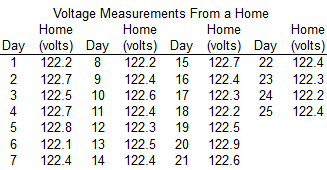Refer to the accompanying data set and use the 25 home voltage measurements to construct a frequency distribution with five classes. Begin with a lower class limit of 122.0 ​volts, and use a class width of 0.2 volt.

Does the result appear to have a normal​ distribution? Why or why​ not?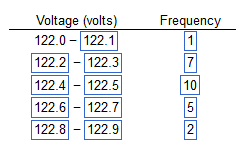Yes, because the frequencies start low, reach a maximum, then become low again, and are roughly symmetric about the maximum frequency.

2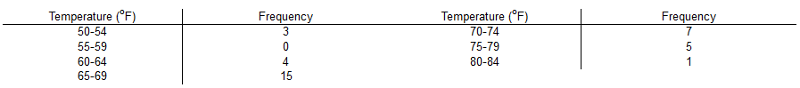Does the frequency distribution appear to have a normal distribution using a strict interpretation of the relevant​ criteria?

No, the distribution does not appear to be normal.

3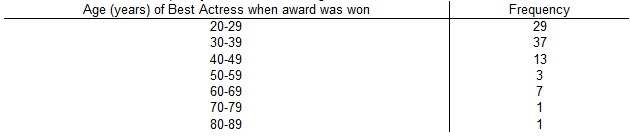Construct the cumulative frequency distribution for the given data.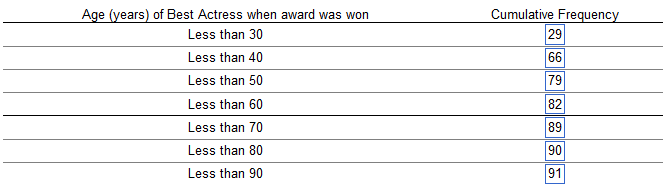4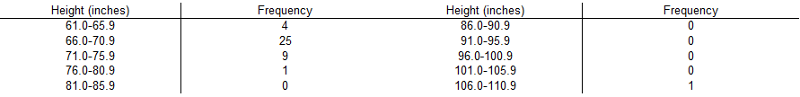What is the class​ width?

What are the class​ midpoints?

What are the class​ boundaries?

5

63.45, 68.45, ..., 103.45, 108.45

60.95, 65.95, ..., 100.95, 105.95, 110.95

5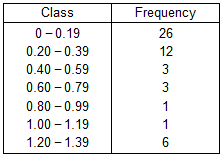The table below shows the frequency distribution of the rainfall on 52 consecutive Wednesdays in a certain city. Use the frequency distribution to construct a histogram.

Do the data appear to have a distribution that is approximately​ normal?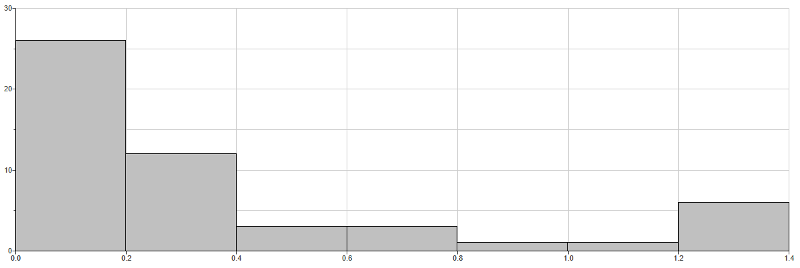No, it is not symmetric.

6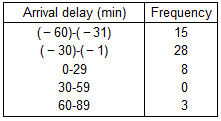The frequency distribution below shows arrival delays for airplane flights.

Use the frequency distribution to construct a histogram. Which part of the histogram depicts flights that arrived​ early, and which part depicts flights that arrived​ late?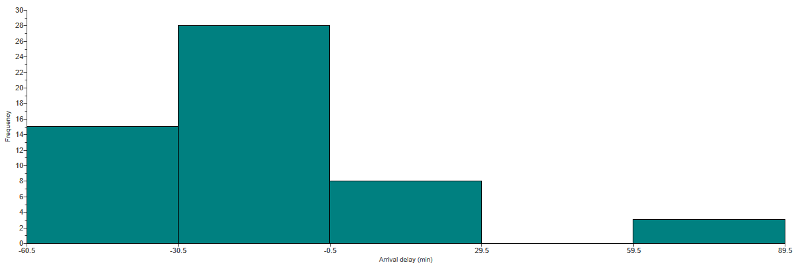The two leftmost bars depict flights that arrived​ early, and the other bars to the right depict flights that arrived late.

7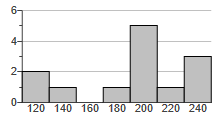The histogram to the right represents the weights​ (in pounds) of members of a certain​ high-school math team.

How many team members are included in the​ histogram?

The histogram represents 13 math team members.

8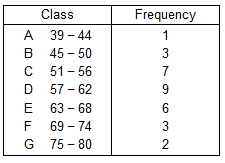The frequency distribution below represents frequencies of actual low temperatures recorded during the course of a​ 31-day month.

Use the frequency distribution to construct a histogram.

Do the data appear to have a distribution that is approximately​ normal?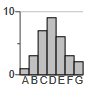Yes, the distribution does appear to be normal.

9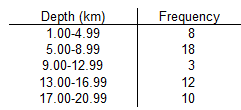The given data represents a frequency distribution of the depth of recent earthquakes. Use the frequency distribution to construct a frequency polygon.

Applying a strict interpretation of the requirements for a normal​ distribution, do the depths appear to be normally​ distributed? Why or why​ not?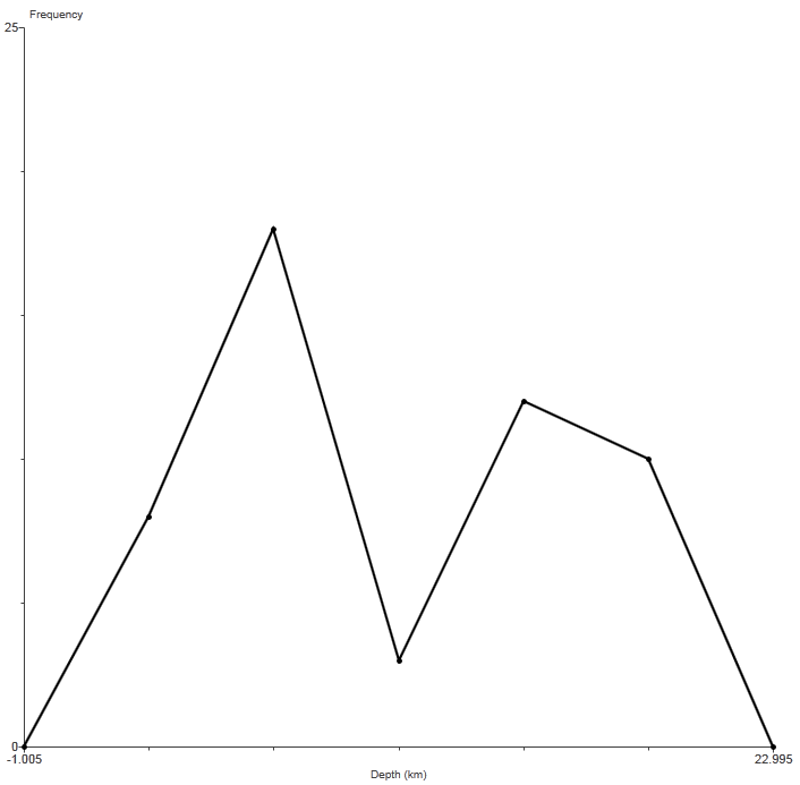The frequency polygon does not appear to approximate a normal distribution because the frequencies do not increase to a maximum and then​ decrease, and the graph is not symmetric.

10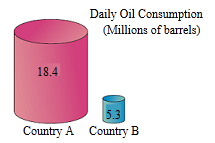The graph to the right uses cylinders to represent barrels of oil consumed by two countries.

Does the graph distort the data? Why or why​ not? If the graph distorts the​ data, construct a graph that depicts the data fairly.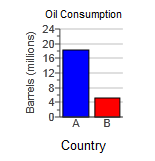Yes, because the graph incorrectly uses objects of volume to represent the data.

11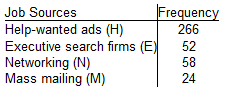A study was conducted to determine how people get jobs. The table lists data from 400 randomly selected subjects.

Construct a Pareto chart that corresponds to the given data.

If someone would like to get a​ job, what seems to be the most effective​ approach?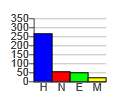12

Which of the following is NOT true about statistical​ graphs?

They utilize areas or volumes for data that are​ one-dimensional in nature.

13

Which of the following is a common distortion that occurs in​ graphs?

Using a​ two-dimensional object to represent data that are​ one-dimensional in nature

14